(zhuan) Building Convolutional Neural Networks with Tensorflow

0
0
01. 云栖社区>
2. 博客>
3. 正文

(zhuan) Building Convolutional Neural Networks with Tensorflow

wangxiaocvpr 2017-08-17 11:18:00 浏览1056

Building Convolutional Neural Networks with Tensorflow

Posted on augustus 15, 2017

1. Introduction

In the past I have mostly written about ‘classical’ Machine Learning, like Naive Bayes classificationLogistic Regression, and the Perceptron algorithm. In the past year I have also worked with Deep Learning techniques, and I would like to share with you how to make and train a Convolutional Neural Network from scratch, using tensorflow. Later on we can use this knowledge as a building block to make interesting Deep Learning applications.

For this you will need to have tensorflow installed (see installation instructions) and you should also have a basic understanding of Python programming and the theory behind Convolutional Neural Networks. After you have installed tensorflow, you can run the smaller Neural Networks without GPU, but for the deeper networks you will definitely need some GPU power.
The Internet is full with awesome websites and courses which explain how a convolutional neural network works. Some of them have good visualisations which make it easy to understand [click here for more info]. I don’t feel the need to explain the same things again, so before you continue, make sure you understand how a convolutional neural network works. For example,

• What is a convolutional layer, and what is the filter of this convolutional layer?
• What is an activation layer (ReLu layer (most widely used), sigmoid activation or tanh)?
• What is a pooling layer (max pooling / average pooling), dropout?
• How does Stochastic Gradient Descent work?

The contents of this blog-post is as follows:

1. Tensorflow basics:
• 1.1 Constants and Variables
• 1.2 Tensorflow Graphs and Sessions
• 1.3 Placeholders and feed_dicts
2. Neural Networks in Tensorflow
• 2.1 Introduction
• 2.3 Creating a (simple) 1-layer Neural Network:
• 2.4 The many faces of Tensorflow
• 2.5 Creating the LeNet5 CNN
• 2.6 How the parameters affect the outputsize of an layer
• 2.7 Adjusting the LeNet5 architecture
• 2.8 Impact of Learning Rate and Optimizer
3. Deep Neural Networks in Tensorflow
• 3.1 AlexNet
• 3.2 VGG Net-16
• 3.3 AlexNet Performance
4. Final words

1. Tensorflow basics:

Here I will give a short introduction to Tensorflow for people who have never worked with it before. If you want to start building Neural Networks immediatly, or you are already familiar with Tensorflow you can go ahead and skip to section 2. If you would like to know more about Tensorflow, you can also have a look atthis repository, or the notes of lecture 1 and lecture 2 of Stanford’s CS20SI course.

1.1 Constants and Variables

The most basic units within tensorflow are Constants, Variables and Placeholders.

The difference between a tf.constant() and a tf.Variable() should be clear; a constant has a constant value and once you set it, it cannot be changed.  The value of a Variable can be changed after it has been set, but the type and shape of the Variable can not be changed.

Besides the tf.zeros() and tf.ones(), which create a Tensor initialized to zero or one (see here), there is also the tf.random_normal() function which create a tensor filled with values picked randomly from a normal distribution (the default distribution has a mean of 0.0 and stddev of 1.0).
There is also the tf.truncated_normal() function, which creates an Tensor with values randomly picked from a normal distribution, where two times the standard deviation forms the lower and upper limit.

With this knowledge, we can already create weight matrices and bias vectors which can be used in a neural network.

1.2. Tensorflow Graphs and Sessions

In Tensorflow, all of the different Variables and the operations done on these Variables are saved in a Graph. After you have build a Graph which contains all of the computational steps necessary for your model, you can run this Graph within a Session. This Session then distributes all of the computations across the available CPU and GPU resources.

1.3 Placeholders and feed_dicts

We have seen the various forms in which we can create constants and variables. Tensorflow also has placeholders; these do not require an initial value and only serve to allocate the necessary amount of memory. During a session, these placeholder can be filled in with (external) data with a feed_dict.

Below is an example of the usage of a placeholder.

2. Neural Networks in Tensorflow

2.1 Introduction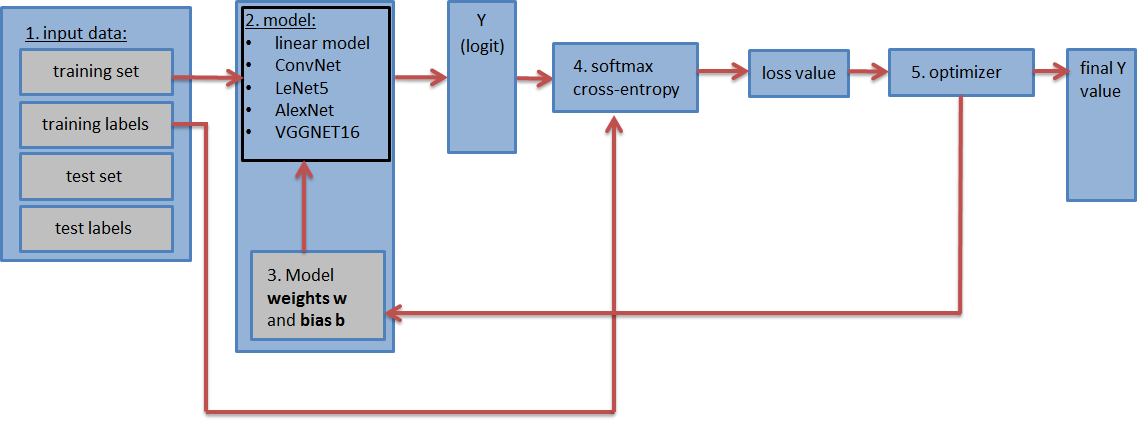The graph containing the Neural Network (illustrated in the image above) should contain the following steps:

1. The input datasets; the training dataset and labels, the test dataset and labels (and the validation dataset and labels).
The test and validation datasets can be placed inside a tf.constant(). And the training dataset is placed in a tf.placeholder() so that it can be feeded in batches during the training (stochastic gradient descent).
2. The Neural Network model with all of its layers. This can be a simple fully connected neural network consisting of only 1 layer, or a more complicated neural network consisting of 5, 9, 16 etc layers.
3. The weight matrices and bias vectors defined in the proper shape and initialized to their initial values. (One weight matrix and bias vector per layer.)
4. The loss value: the model has as output the logit vector (estimated training labels) and by comparing the logit with the actual labels, we can calculate the loss value (with the softmax with cross-entropy function). The loss value is an indication of how close the estimated training labels are to the actual training labels and will be used to update the weight values.
5. An optimizer, which will use the calculated loss value to update the weights and biases with backpropagation.

Let’s load the dataset which are going to be used to train and test the Neural Networks. For this we will download the MNIST and the CIFAR-10 dataset. The MNIST dataset contains 60.000 images of handwritten digits, where each image size is 28 x 28 x 1 (grayscale). The CIFAR-10 dataset contains 60.000 colour images (3 channels) – size 32 x 32 x 3 – of 10 different objects (airplane, automobile, bird, cat, deer, dog, frog, horse, ship, truck). Since there are 10 different objects in each dataset, both datasets contain 10 labels.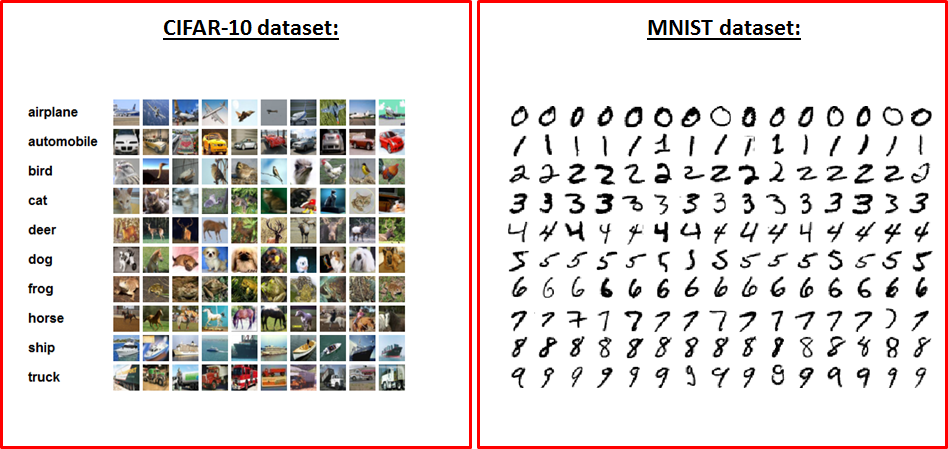First, lets define some methods which are convenient for loading and reshaping the data into the necessary format.

These are methods for one-hot encoding the labels, loading the data in a randomized array and a method for flattening an array (since a fully connected network needs an flat array as its input):

After we have defined these necessary function, we can load the MNIST and  CIFAR-10 datasets with:

You can download the MNIST dataset from Yann LeCun’s website.  After you have downloaded and unzipped the files, you can load the data with the python-mnist tool. CIFAR-10 can be downloaded from here.

2.3 Creating a (simple) 1-layer Neural Network

The most simple form of a Neural Network is a 1-layer linear Fully Connected Neural Network (FCNN). Mathematically it consists of a matrix multiplication.
It is best to start with such a simple NN in tensorflow, and later on look at the more complicated Neural Networks. When we start looking at these more complicated Neural Networks, only the model (step 2) and weights (step 3) part of the Graph will change and the other steps will remain the same.

We can make such an 1-layer FCNN as follows:

This is all there is too it! Inside the Graph, we load the data, define the weight matrices and the model, calculate the loss value from the logit vector and pass this to the optimizer which will update the weights for ‘num_steps’ number of iterations.

In the above fully connected NN, we have used the Gradient Descent Optimizer for optimizing the weights. However, there are many different optimizers available in tensorflow. The most common used optimizers are the GradientDescentOptimizer, AdamOptimizer and AdaGradOptimizer, so I would suggest to start with these if youre building a CNN.
Sebastian Ruder has a nice blog post explaining the differences between the different optimizers which you can read if you want to know more about them.

2.4 The many faces of Tensorflow

Tensorflow contains many layers, meaning the same operations can be done with different levels of abstraction. To give a simple example, the operation
logits = tf.matmul(tf_train_dataset, weights) + biases,
can also be achieved with
logits = tf.nn.xw_plus_b(train_dataset, weights, biases).

This is the best visible in the layers API, which is an layer with a high level of abstraction and makes it very easy to create Neural Network consisting of many different layers. For example, the conv_2d() or thefully_connected() functions create convolutional and fully connected layers. With these functions, the number of layers, filter sizes / depths, type of activation function, etc can be specified as a parameter. The weights and bias matrices are then automatically created, as well as the additional activation functions and dropout regularization layers.

For example, with the layers API, the following lines:

can be replaced with

As you can see, we don’t need to define the weights, biases or  activation functions. Especially when youre building a neural network with many layers, this keeps the code succint and clean.

However, if youre just starting out with tensorflow and want to learn how to build different kinds of Neural Networks, it is not ideal, since were letting tflearn do all the work.
Therefore we will not use the layers API in this blog-post, but I do recommend you to use it once you have a full understanding of how a neural network should be build in tensorflow.

2.5 Creating the LeNet5 CNN

Let’s start with building more layered Neural Network.  For example the LeNet5 Convolutional Neural Network.

The LeNet5 CNN architecture was thought of by Yann Lecun as early as in 1998 (see paper). It is one of the earliest CNN’s (maybe even the first?) and was specifically designed to classify handwritten digits. Although it performs well on the MNIST dataset which consist of grayscale images of size 28 x 28, the performance drops on other datasets with more images, with a larger resolution (larger image size) and more classes. For these larger datasets, deeper ConvNets (like AlexNet, VGGNet or ResNet), will perform better.

But since the LeNet5 architecture only consists of 5 layers, it is a good starting point for learning how to build CNN’s.

The Lenet5 architecture looks as follows: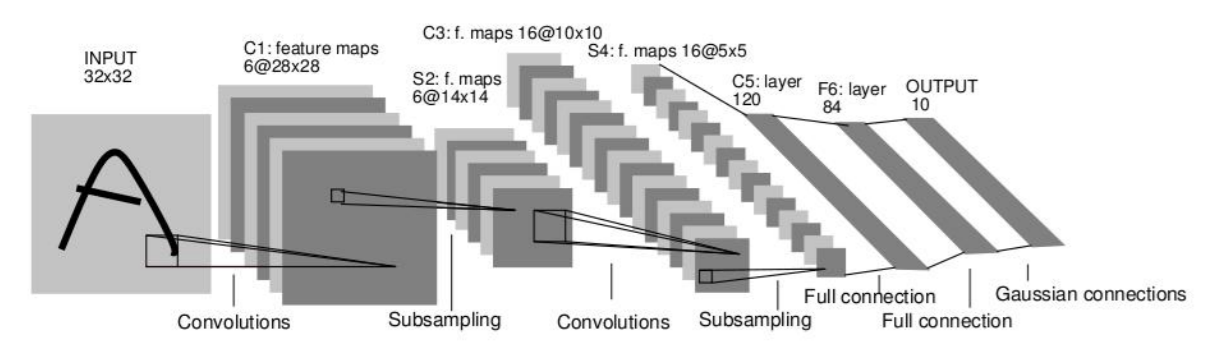As we can see, it consists of 5 layers:

• layer 1: a convolutional layer, with a sigmoid activation function, followed by an average pooling layer.
• layer 2: a convolutional layer, with a sigmoid activation function, followed by an average pooling layer.
• layer 3: a fully connected network (sigmoid activation)
• layer 4: a fully connected network (sigmoid activation)
• layer 5: the output layer

This means that we need to create 5 weight and bias matrices, and our model will consists of 12 lines of code (5 layers + 2 pooling + 4 activation functions + 1 flatten layer).
Since this is quiet some code, it is best to define these in a seperate function outside of the graph.

With the variables, and model defined seperately, we can adjust the the graph a little bit so that it uses these weights and model instead of the previous Fully Connected NN:

As we can see the LeNet5 architecture performs better on the MNIST dataset than a simple fully connected NN.

2.6 How the parameters affect the outputsize of an layer

Generally it is true that the more layers a Neural Network has, the better it performs. We can add more layers, change activation functions and pooling layers, change the learning rate and see how each step affects the performance. Since the input of layeris the output of layer, we need to know how the output size of layeris affected by its different parameters.

To understand this, lets have a look at the conv2d() function.

It has four parameters:

• The input image, a 4D Tensor with dimensions [batch size, image_width, image_height, image_depth]
• An weight matrix, a 4-D Tensor with dimensions [filter_size, filter_size, image_depth, filter_depth]
• The number of strides in each dimension.
• Padding (= ‘SAME’ / ‘VALID’)

These four parameters determine the size of the output image.

The first two parameters are the 4-D Tensor containing the batch of input images and the 4-D Tensor containing the weights of the convolutional filter.

The third parameter is the stride of the convolution, i.e. how much the convolutional filter should skip positions in each of the four dimension. The first of these 4 dimensions indicates the image-number in the batch of images and since we dont want to skip over any image, this will always be 1. The last dimension indicates the image depth (no of color-channels; 1 for grayscale and 3 for RGB) and since we dont want to skip over any color-channels, this is also always 1. The second and  third dimension indicate the stride in the X and Y direction (image width and height).  If we want to apply a stride, these are the dimensions in which the filter should skip positions. So for a stride of 1, we have to set the stride-parameter to [1, 1, 1, 1] and if we want a stride of 2, set it to [1, 2, 2, 1]. etc

The last parameter indicates whether or not tensorflow should zero-pad the image in order to make sure the output size does not change size for a stride of 1. With padding = ‘SAME’ the image does get zero-padded (and output size does not change), with padding = ‘VALID’ it does not.

Below we can see two examples of a convolutional filter (with filter size 5 x 5) scanning through an image (of size 28 x 28).
On the left the padding parameter is set to ‘SAME’, the image is zero-padded and the last 4 rows / columns are included in the output image.
On the right padding is set to ‘VALID’, the image does not get zero-padded and the last 4 rows/columns are not included.

GIF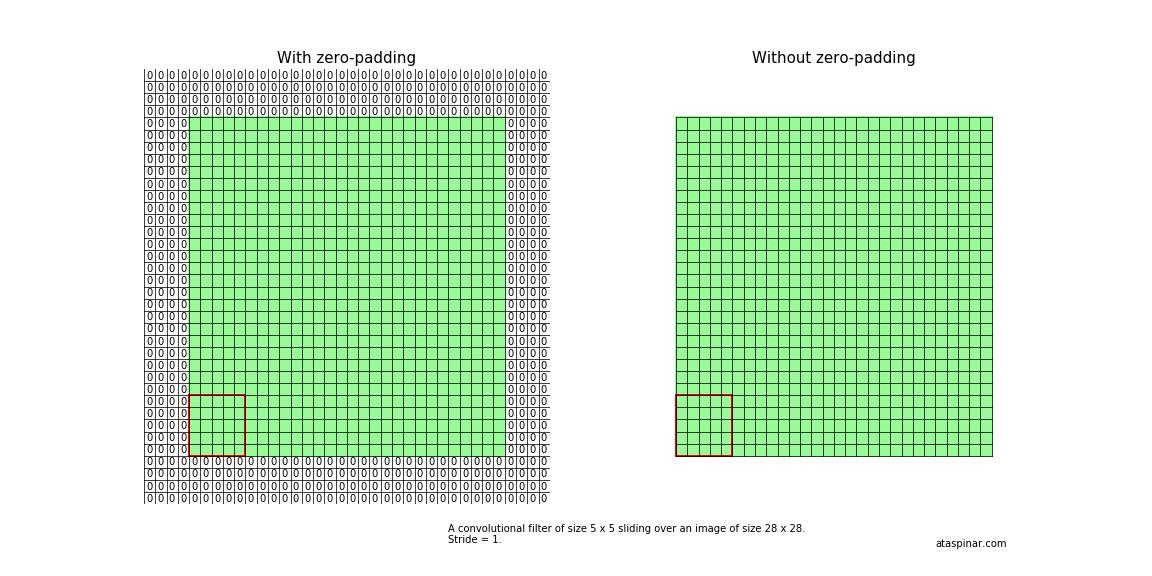As we can see, without zero-padding the last four cells are not included, because the convolutional filter has reached the end of the (non-zero padded) image. This means that, for an input size of 28 x 28, the output size becomes 24 x 24. If padding = ‘SAME’,  the output size is 28 x 28.

This becomes more clear if we write down the positions of the filter on the image while it is scanning through the image (For simplicity, only the X-direction). With a stride of 1, the X-positions are 0-5, 1-6, 2-7, etc. If the stride is 2, the X-positions are 0-5, 2-7, 4-9, etc.

If we do this for an image size of 28 x 28, filter size of 5 x 5 and strides 1 to 4, we will get the following table: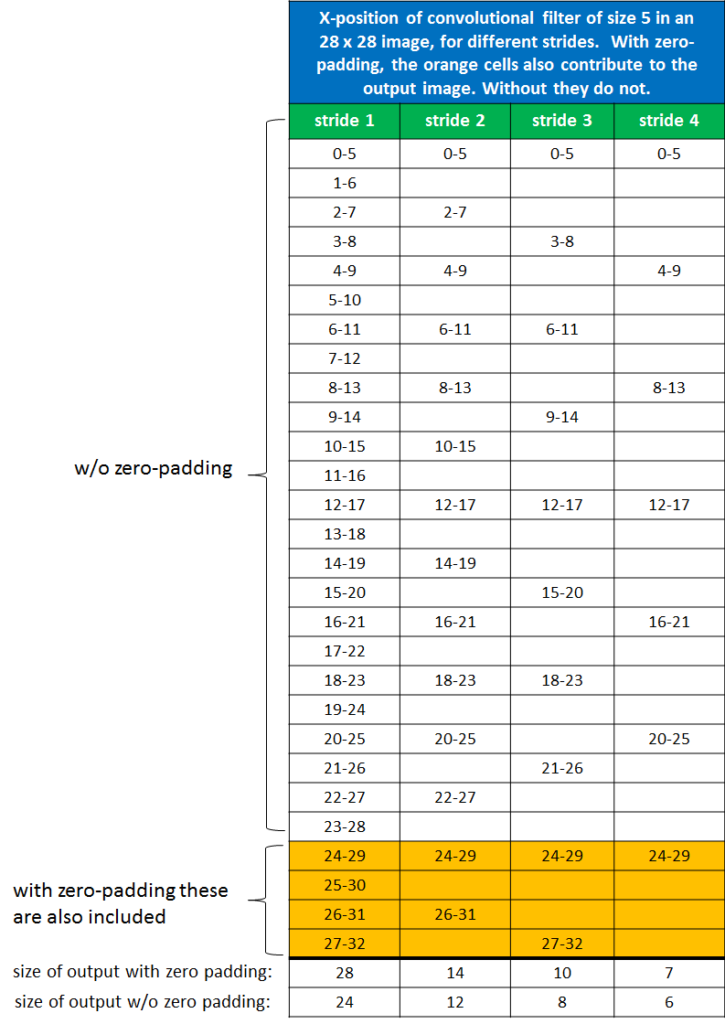As you can see, for a stride of 1, and zero-padding the output image size is 28 x 28. Without zero-padding the output image size becomes 24 x 24. For a filter with a stride of 2, these numbers are 14 x 14 and 12 x 12, and for a filter with stride 3 it is 10 x 10 and 8 x 8. etc

For any arbitrary chosen stride S, filter size K, image size W, and padding-size P, the output size will beIf padding = ‘SAME’ in tensorflow, the numerator always adds up to 1 and the output size is only determined by the stride S.

2.7 Adjusting the LeNet5 architecture

In the original paper, a sigmoid activation function and average pooling were used in the LeNet5 architecture. However, nowadays, it is much more common to use a relu activation function. So let’s change the LeNet5 CNN a little bit to see if we can improve its accuracy. We will call this the LeNet5-like Architecture:

The main differences are that we are using a relu activation function instead of a sigmoid activation.

Besides the activation function, we can also change the used optimizers to see what the effect is of the different optimizers on accuracy.

2.8 Impact of Learning Rate and Optimizer

Lets see how these CNN’s perform on the MNIST and CIFAR-10 datasets.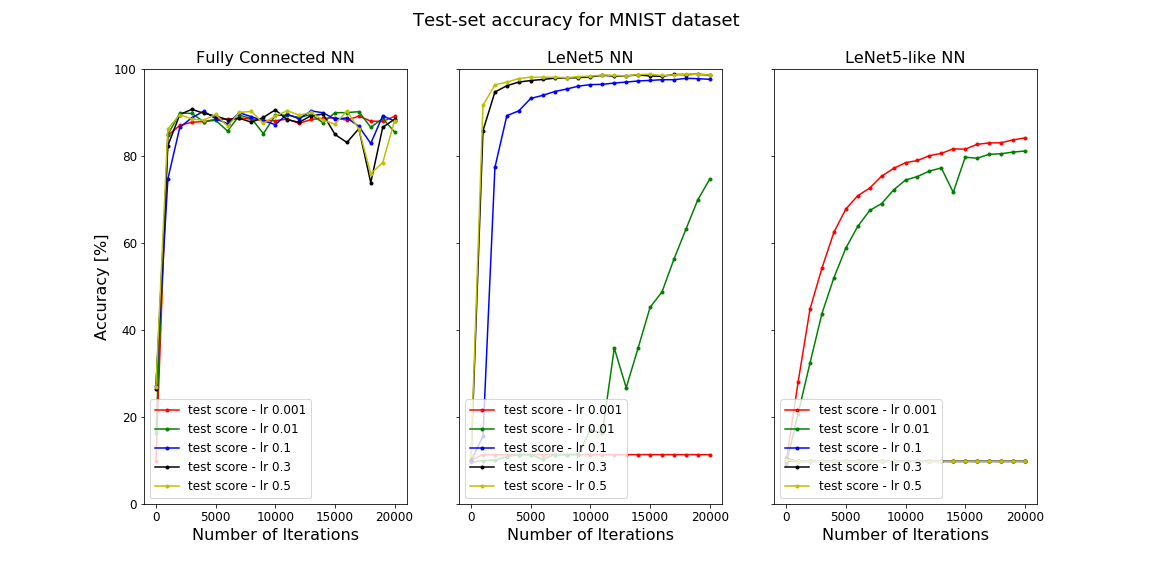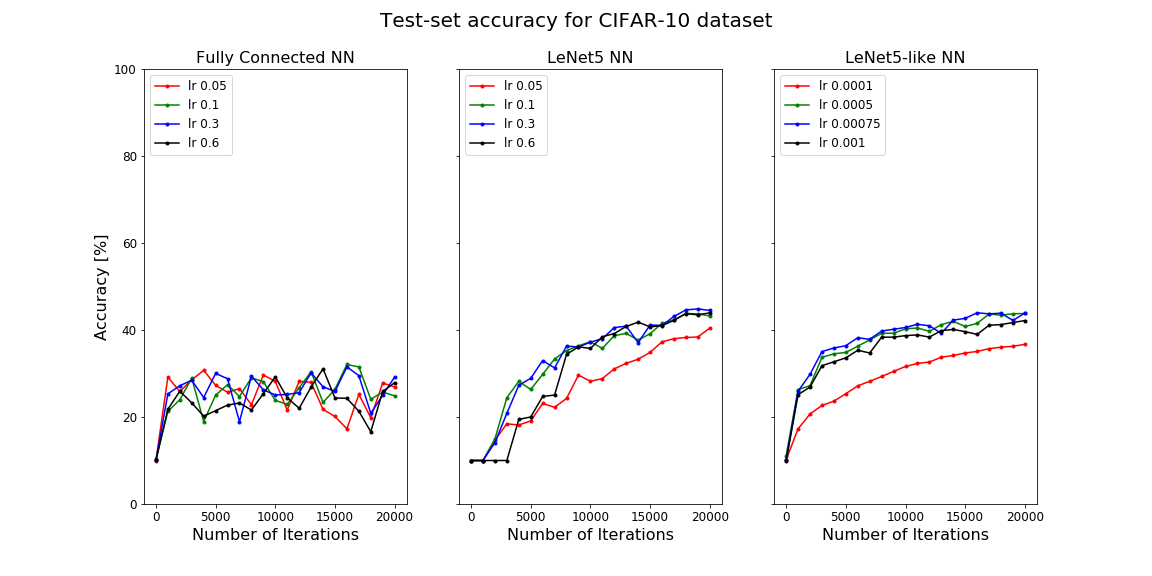In the figures above, the accuracy on the test set is given as a function of the number of iterations. On the left for the one layer fully connected NN, in the middle for the LeNet5 NN and on the right for the LeNet5-like NN.

As we can see, the LeNet5 CNN works pretty good for the MNIST dataset. Which should not be such a big surprise, since it was specially designed to classify handwritten digits. The MNIST dataset is quiet small and does not provide a big challenge, so even a one layer fully connected network performs quiet good.

On the CIFAR-10 Dataset however, the performance for the LeNet5 NN drops significantly to accuracy values around 40%.

To increase the accuracy, we can change the optimizer, or fine-tune the Neural Network by applying regularization or learning rate decay.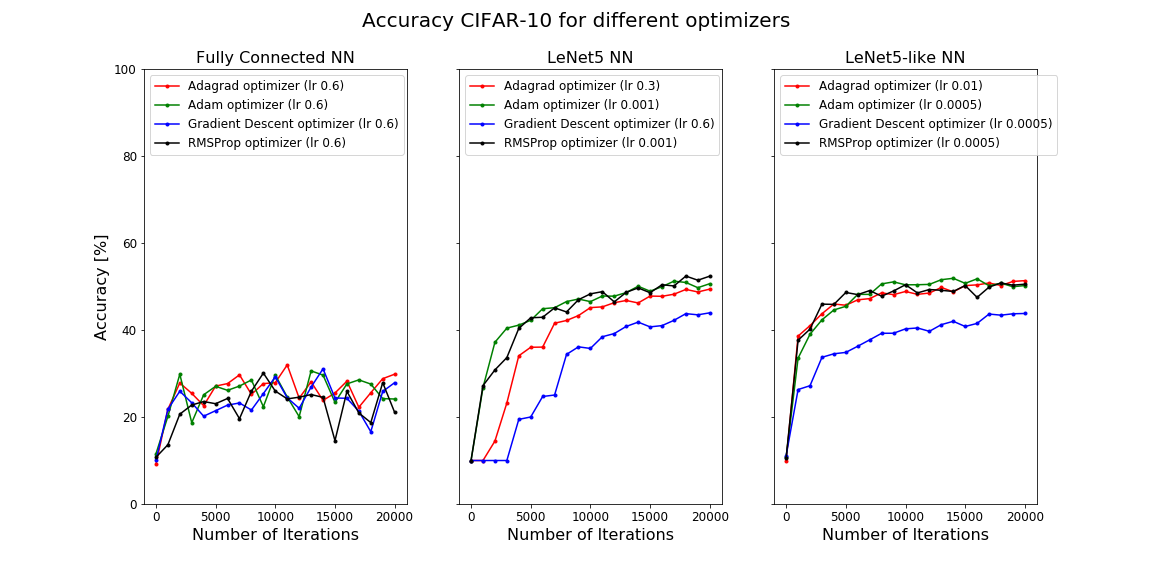As we can see, the AdagradOptimizer, AdamOptimizer and the RMSPropOptimizer have a better performance than the GradientDescentOptimizer. These are adaptive optimizers which in general perform better than the (simple) GradientDescentOptimizer but need more computational power.

With L2-regularization or exponential rate decay we can probably gain a bit more accuracy, but for much better results we need to go deeper.

3. Deep Neural Networks in Tensorflow

So far we have seen the LeNet5 CNN architecture. LeNet5 contains two convolutional layers followed by fully connected layers and therefore could be called a shallow Neural Network. At that time (in 1998) GPU’s were not used for computational calculations, and the CPU’s were not even that powerful so for that time the two convolutional layers were already quiet innovative.

Later on, many other types of Convolutional Neural Networks have been designed, most of them much deeper [click here for more info].
There is the famous AlexNet architecture (2012) by  Alex Krizhevsky et. al., the 7-layered ZF Net (2013), and the 16-layered VGGNet (2014).
In 2015 Google came with 22-layered CNN with an inception module (GoogLeNet), and Microsoft Research Asia created the 152-layered CNN called ResNet.

Now, with the things we have learned so far, lets see how we can create the AlexNet and VGGNet16 architectures in Tensorflow.

3.1 AlexNet

Although LeNet5 was the first ConvNet, it is considered to be a shallow neural network. It performs well on the MNIST dataset which consist of grayscale images of size 28 x 28, but the performance drops when we’re trying to classify larger images, with more resolution and more classes.

The first Deep CNN came out in 2012 and is called AlexNet after its creators Alex Krizhevsky, Ilya Sutskever, and Geoffrey Hinton. Compared to the most recent architectures AlexNet can be considered simple, but at that time it was really succesfull. It won the ImageNet competition with a incredible test error rate of 15.4% (while the runner-up had an error of 26.2%) and started a revolution (also see this video) in the world of Deep Learning and AI.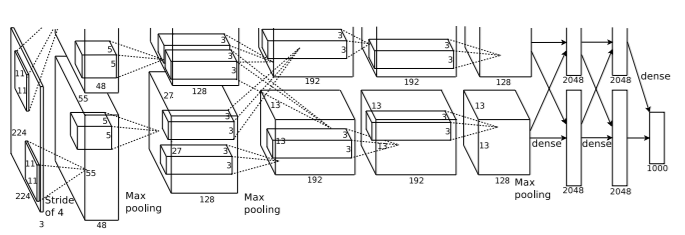It consists of 5 convolutional layers (with relu activation), 3 max pooling layers, 3 fully connected layers and 2 dropout layers. The overall architecture looks as follows:

• layer 0: input image of size 224 x 224 x 3
• layer 1: A convolutional layer with 96 filters (filter_depth_1 = 96) of size 11 x 11 (filter_size_1 = 11) and a stride of 4. It has a relu activation function.
This is followed by max pooling and local response normalization layers.
• layer 2: A convolutional layer with 256 filters (filter_depth_2 = 256) of size 5 x 5 (filter_size_2 = 5) and a stride of 1. It has a relu activation function.
This layer is also followed by max pooling and local response normalization layers.
• layer 3: A convolutional layer with 384 filters (filter_depth_3 = 384) of size 3 x 3 (filter_size_3 = 3) and a stride of 1. It has a relu activation function.
• layer 4: Same as layer 3.
• layer 5: A convolutional layer with 256 filters (filter_depth_4 = 256) of size 3 x 3 (filter_size_4 = 3) and a stride of 1. It has a relu activation function.
• layer 6-8: These convolutional layers are followed by fully connected layers with 4096 neurons each. In the original paper they are classifying a dataset with 1000 classes, but we will use the oxford17 dataset, which has 17 different classes (of flowers).

Note that this CNN (or other deep CNN’s) cannot be used on the MNIST or the CIFAR-10 dataset, because the images in these datasets are too small. As we have seen before, a pooling layer (or a convolutional layer with a stride of 2) reduces the image size by a factor of 2. AlexNet has 3 max pooling layers and one convolutional layer with a stride of 4. This means that the original image size gets reduced by a factor of. The images in the MNIST dataset would simply get reduced to a size smaller than 0.

Therefore we need to load a dataset with larger images, preferably 224 x 224 x 3 (as the original paper indicates). The 17 category flower dataset, aka oxflower17 dataset is ideal since it contains images of exactly this size:

Lets try to create the weight matrices and the different layers present in AlexNet. As we have seen before, we need as much weight matrices and bias vectors as the amount of layers, and each weight matrix should have a size corresponding to the filter size of the layer it belongs to.

Now we can modify the CNN model to use the weights and layers of the AlexNet model in order to classify images.

3.2 VGG Net-16

VGG Net was created in 2014 by Karen Simonyan and Andrew Zisserman of the University of Oxford. It contains much more layers (16-19 layers), but each layer is simpler in its design; all of the convolutional layers have filters of size 3 x 3 and stride of 1 and all max pooling layers have a stride of 2.
So it is a deeper CNN but simpler.

It comes in different configurations, with either 16 or 19 layers. The difference between these two different configurations is the usage of either 3 or 4 convolutional layers after the second, third and fourth max pooling layer (see below).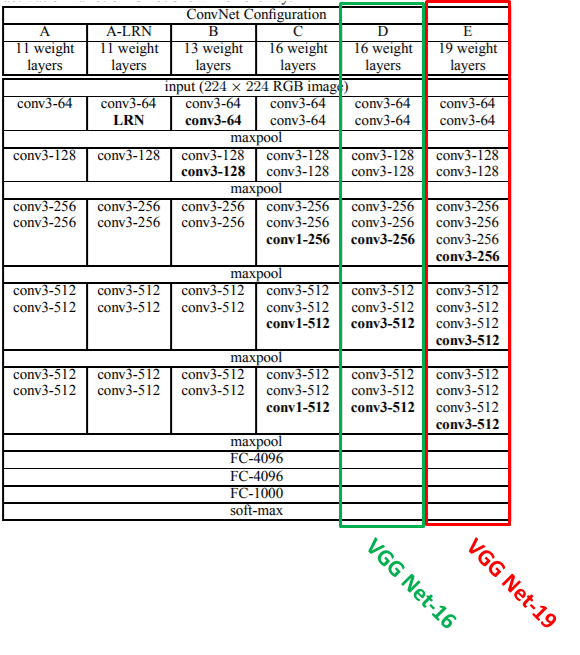The configuration with 16 layers (configuration D) seems to produce the best results, so lets try to create that in tensorflow.

3.3 AlexNet Performance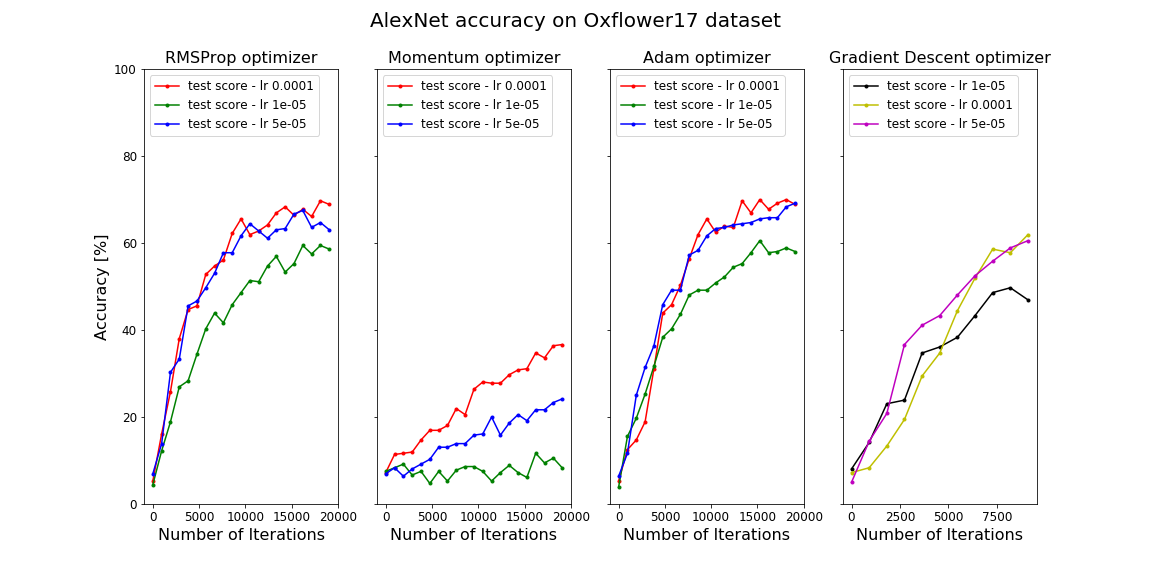As a comparison, have a look at the LeNet5 CNN performance on the larger oxflower17 dataset:

4. Final Words

The code is also available in my GitHub repository, so feel free to use it on your own dataset(s).

There is much more to explore in the world of Deep Learning; Recurrent Neural Networks, Region-Based CNN’s, GAN’s, Reinforcement Learning, etc. In future blog-posts I’ll build these types of Neural Networks, and also build awesome applications with what we have already learned.
So subscribe and stay tuned!

 If you feel like you need to refresh your understanding of CNN’s, here are some good starting points to get you up to speed:

 If you want more information about the theory behind these different Neural Networks, Adit Deshpande’s blog post provides a good comparison of them with links to the original papers. Eugenio Culurciello has a nice blog and article worth a read.  In addition to that, also have a look at this github repository containing awesome deep learning papers, and this github repository where deep learning papers are ordered by task and date.